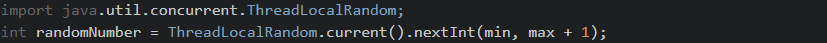# Java - Generating random integersJava, random

While generating random int values in a specific range is fairly simple, it still requires that you understand the syntax and how to use the Java Random class. Let's take a quick look at some examples below which go over some options when generating a random number in Java depending on your Java version.

### Java 1.6 and older

In older version of java it is pretty standard to use the java.util.Random class. This is preferable to using the java.lang.Math.random() because why reinvent the wheel? Use the standard library!

``import `java.util.Random;public static int randomInteger(int min, int max) { Random rand; int randomNumber = rand.nextInt((max-min) + 1) + min; return randomNumber;}`

``import `java.util.concurrent.ThreadLocalRandom;int randomNumber = ThreadLocalRandom.current().nextInt(min, max + 1);`